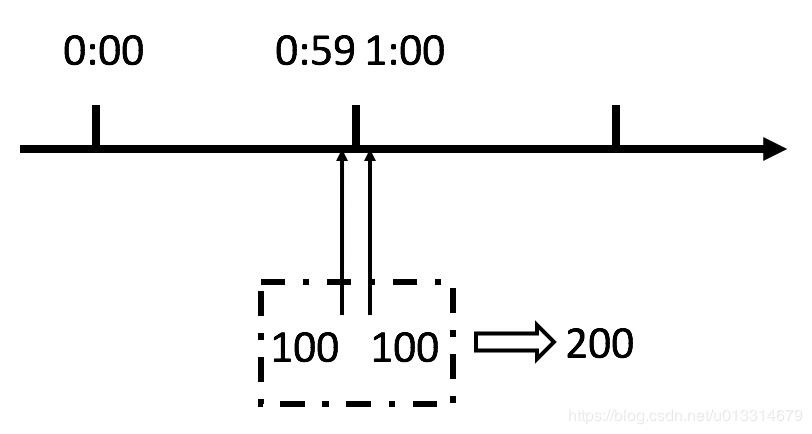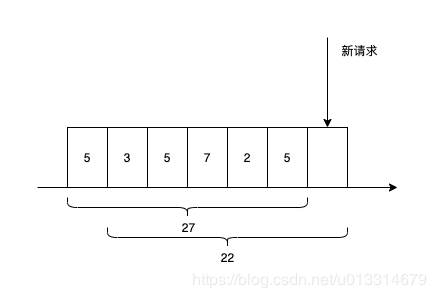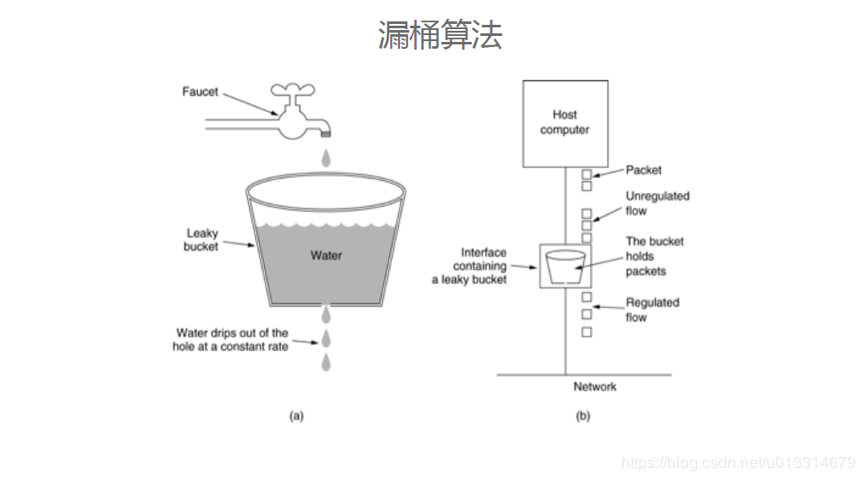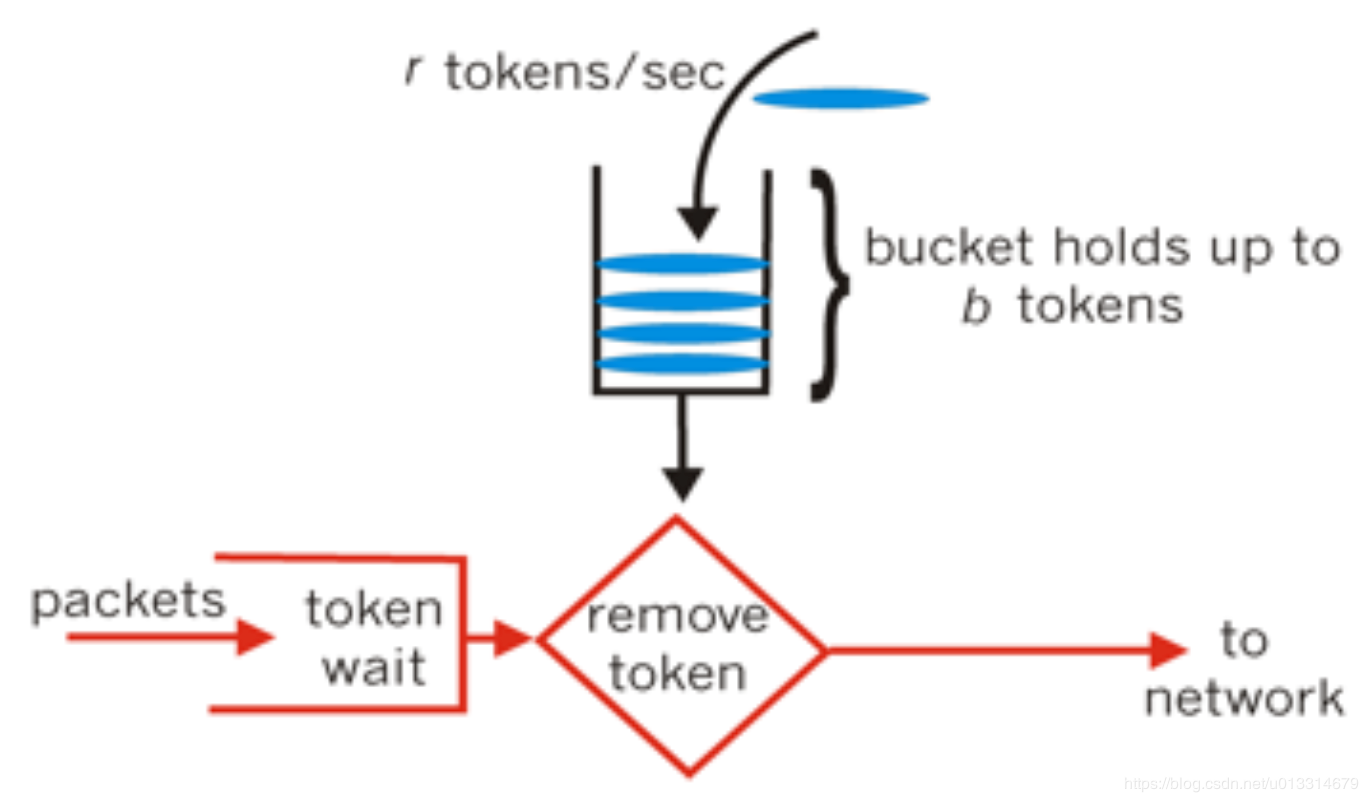# 保障服务稳定之服务限流X先生

﻿

﻿

## 二、解决的问题

﻿

﻿

• 突发热点事件（例如微博热搜）

• 爬虫

• 恶意攻击

• 恶意刷单（如12306）

···

﻿

﻿

## 三、限流算法

### 1、计数器算法

﻿

#### 固定窗口

﻿

PHP实现逻辑如下:

/** * 固定窗口计数器限流算法 * @param $key string 限流依据，例如uid，url等 * @param$time int 限流时间段，单位秒 * @param $limit int 限流总数 */function limit($key, $time,$limit) {	//当前时间所在分片	$current_segment=floor(time() /$time);	//按当前时间和限流参数生成key	$current_key =$key . '_' . $time . '_' .$limit . '_' . $current_segment;$redis = new Redis();	//key不存在才设置，且设置过期时间	$redis->set($current_key, 0, ['nx', 'ex' => $time]);$current = $redis->incr($current_key);	//为了解决请求并发的问题，代码实现上要先加再判断	if ($current >$limit) {		return false;	}	return true;}

﻿

﻿

﻿﻿

#### 滑动窗口

﻿

• 划分多个小的时间段，各时间段各自进行计数。

• 根据当前时间，动态往前滑动来计算时间窗口范围，合并计算总数。﻿

﻿

PHP实现逻辑如下:

/** * 滑动窗口计数器限流算法 * @param $key string 限流依据，例如uid，url等 * @param$time int 限流时间段，单位秒 * @param $limit int 限流总数 * @param$segments_num int 分段个数 */function limit($key,$time, $limit,$segments_num) {	//小分片时间长度	$segments_time=floor($time/$segments_num); //当前时间所在小分片$current_segment=floor(time() / $segments_time); //按限流时间段生成key$current_key = $key . '_' .$time . '_' . $limit . '_' .$current_segment;		$redis = new Redis(); //先更新当前时间所在小分片计数，key不存在才设置，且设置过期时间$redis->set($current_key, 0, ['nx', 'ex' =>$time]);	$current =$redis->incr($current_key); for($window=$segments_time;$window<$time;$window+=$segments_time){$current_segment=$current_segment-1;$tmp_key = $key . '_' .$time . '_' . $limit . '_' .$current_segment;		//计算时间窗口内的总数		$current+=intval($redis->get($tmp_key)); if ($current > $limit) { //超过限制的话要回滚本次加的次数$redis->decr($current_key); return false; } } return true;} ﻿ 缺点 ﻿ 滑动窗口限流算法虽然可以保证任意时间窗口内接口请求次数都不会超过最大限流值，但是相对来说对系统的瞬时处理能力还是没有考虑到，无法防止在更细的时间粒度上访问过于集中的问题，例如在同一时刻（同一秒）大量请求涌入，还是可能会超过系统负荷能力。 ﻿ ### 2、漏桶算法 漏桶算法就是一种从系统的处理能力出发进行限流的算法。类似生活用到的漏斗，上面进水，下面有个小口出水，当请求进来时，相当于水倒入漏斗，然后从下端小口慢慢匀速的流出。不管上面流量多大，下面流出的速度始终保持不变，超过漏斗容量的则丢弃。漏桶算法以固定的速率释放访问请求（即请求通过），直到漏桶为空。漏桶算法有两个关键数据：桶的容量V和流出的速率R。假设每个请求的平均处理时间是S，最大超时时间是SS，则V/R+S<=SS。 ﻿ 可以使用队列来实现，队列设置最大容量，访问请求先进入队列，队列满了的话就丢弃丢弃后续请求，然后通过另外一个woker以固定速率从队列出口拿请求去处理。具体实现逻辑不展示了。 ﻿ 缺点 漏桶算法的缺陷很明显，由于出口的处理速率是固定的，当短时间内有大量的突发请求时，即便此时服务器没有任何负载，每个请求也都得在队列中等待一段时间才能被响应，因此漏桶算法无法应对突发流量。 ﻿ ### 3、令牌桶算法 令牌桶算法也是有一个桶，但是它不是通过限制漏出的请求来控制流量，而是通过控制桶的令牌的生成数量来达到限流的目的的。令牌桶定时往桶里面丢一定的令牌，令牌桶满了就不再往里面加令牌。每来一个请求就要先在桶里拿一个令牌，拿到令牌则通过，拿不到则拒绝。 ﻿﻿ 当访问量小于令牌桶的生成速率时，令牌桶可以慢慢积累令牌直到桶满，这样当短时间的突发访问量来时，其积累的令牌数保证了大量请求都能立刻拿到令牌进行后续处理。当访问量持续大量流入时，积累的令牌被消耗完了之后，后续请求又依赖于一定速率产生的新令牌，这时候就变成类似漏桶算法那样的固定流量限制。 ﻿ 由此可见，相比于漏桶算法，令牌桶算法能够在限制调用的平均速率的同时还允许一定程度的突发调用。 ﻿ PHP实现逻辑如下: /** * 令牌桶限流算法 * @param$key string 限流依据，例如uid，url等 * @param $rate float 令牌生成速率，每秒$rate个 * @param $volume int 容量 * @return bool */function limit($key, $rate,$volume) {	//按限流参数生成key	$current_key =$key . '_' . $rate . '_' .$volume;	$redis = new Redis();$time=time();		//没有则初始化	$redis->hSetNx($current_key, 'num', $volume);$redis->hSetNx($current_key, 'time',$time);	//以下逻辑在高并发情况下要用lua脚本或者加分布式锁，这里仅用于说明算法的逻辑，就不考虑并发情况了	//计算从上次到现在，需要添加的令牌数量	$last=$redis->hMGet($current_key,['num','time']);$last_time=$last['time'];$last_num=$last['num'];$incr=($time-$last_time)*$rate;$current=min($volume,($last_num+$incr));//计算当前令牌数 if($current>0){		$redis->hMSet($current_key,[			'num'=>\$current-1,			'time'=>time()		]);		return true;	}		return false;}## 评论